## research papersJOURNAL OFSYNCHROTRONRADIATION
ISSN: 1600-5775
Volume 25| Part 3| May 2018| Pages 729-737

## Characterization of the surface contamination of deep X-ray lithography mirrors exposed to synchrotron radiation

(Received 9 September 2017; accepted 27 March 2018; online 24 April 2018)

In deep X-ray lithography (DXRL), synchrotron radiation is applied to pattern polymer microstructures. At the Synchrotron Laboratory for Micro and Nano Devices (SyLMAND), Canadian Light Source, a chromium-coated grazing-incidence X-ray double-mirror system is applied as a tunable low-pass filter. In a systematic study, the surface conditions of the two mirrors are analyzed to determine the mirror reflectivity for DXRL process optimization, without the need for spectral analysis or surface probing: PMMA resist foils were homogeneously exposed and developed to determine development rates for mirror angles between 6 mrad and 12 mrad as well as for white light in the absence of the mirrors. Development rates cover almost five orders of magnitude for nominal exposure dose (deposited energy per volume) values of 1 kJ cm−3 to 6 kJ cm−3. The rates vary from case to case, indicating that the actual mirror reflectivity deviates from that of clean chromium assumed for the experiments. Fitting the mirror-based development rates to the white-light case as a reference, reflectivity correction factors are identified, and verified by experimental and numerical results of beam calorimetry. The correction factors are related to possible combinations of a varied chromium density, chromium oxidation and a carbon contamination layer. The best fit for all angles is obtained assuming 7.19 g cm−3 nominal chromium density, 0.5 nm roughness for all involved layers, and an oxide layer thickness of 25 nm with a carbon top coat of 50 nm to 100 nm. A simulation tool for DXRL exposure parameters was developed to verify that the development rates for all cases do coincide within a small error margin (achieving a reduction of the observed errors by more than two orders of magnitude) if the identified mirror surface conditions are considered when calculating the exposure dose.

### 1. Introduction

Synchrotron radiation is a powerful and widely used tool for the analysis of matter. It can also be applied to modify matter. The best known synchrotron-based technique to pattern polymers is deep X-ray lithography (DXRL) and subsequent processes (Becker et al., 1986). Compared with competing lithographic techniques, synchrotron radiation penetrates deep into the material and is naturally collimated. DXRL therefore excels in the fabrication of microstructures with large vertical dimensions, usually ranging from tens to hundreds of micrometers, with very parallel, vertical and optically smooth sidewalls. Typical applications include micro-optics (Mohr et al., 1997), electrostatics (Klymyshyn et al., 2010) and mechanical devices (Meyer et al., 2008). Unlike many synchrotron beamlines used for analyses, DXRL beamlines do not require monochromatic radiation. Excess high-energy components, however, may lead to the detrimental impact of unwanted secondary electrons (Pantenburg & Mohr, 1995), reducing the effective DXRL mask absorber opacity, and increasing the heat load on the overall sample setup (Achenbach et al., 2003). Advanced DXRL beamlines therefore often apply grazing-incidence mirrors as low-pass filters (Megtert et al., 1999), with the most common spectral range used for patterning being 2 keV to 10 keV photons.

SyLMAND, the Synchrotron Laboratory for Micro and Nano Devices at the Canadian Light Source, is a new DXRL laboratory that applies a double-mirror system to allow for a wide range of spectral tuning (Achenbach et al., 2009). Silicon mirror bodies of length 900 mm are coated with 120 nm of chromium. The surface roughness was measured to not exceed 0.5 nm. During scientific commissioning, the beamline, mask and sample conditions were modeled with the DXRL simulation tools DoseSim (Meyer et al., 2002) and Lex-D [described by Griffiths (2004)] to determine the required exposure parameters for different sample cases, including mirror grazing-incidence angles, optimized high-pass pre-filters, and the required energy deposition per volume (referred to as dose'). Test exposures into poly(methyl methacrylate) (PMMA) resist were performed based on these parameters. Development rates in exposed resist were significantly lower than simulated, which corresponds to a much lower dose deposition than anticipated. This discrepancy between simulation and experiment was not observed when the mirrors were moved out of the beam. Therefore, the actual mirror reflectivity must have been much lower than consistently predicted by the simulation tools.

Four parameters can fundamentally impact the mirror reflectivity: (1) the surface material and its roughness, (2) the incidence angle and slope errors, (3) the material density, and (4) surface contaminants.

For (1), the chromium material and its surface roughness were measured prior to mirror installation, and these parameters were not further examined in this study. For (2), the grazing-incidence angles were precisely verified using SyLMAND's in-mirror beam position monitoring (Subramanian et al., 2009) as well as position measurement in the scanner experimental endstation, such that angular offsets can be eliminated. The bending radius of the mirror was measured prior to installation and exceeds 40 km. Thermal deformation under beam has previously been reported at other facilities, but is very unlikely to play a role in this study: first, even if the mirror warped by up to 5 mrad no further aperture-driven collimation of the beam would occur under the experimental conditions; second, the same results are observed even when the incident beam power is reduced by one to two orders of magnitude using the collimating slits upstream and the intensity chopper. Slope errors and thermal deformations are therefore not further considered. For (3), the density of the sputter-deposited chromium was never measured and could well divert from the textbook value of 7.19 g cm−3. Furthermore, for (4), the chromium surface could potentially have become oxidized during mirror installation or beamline upgrades. Even upon synchrotron exposure, chromium mirrors form a native oxide layer of several nanometers thickness (Andreeva, 1964; Sandberg et al., 2003), which reduces the mirror reflectivity compared with an ideal chromium surface (Michette, 1986). Nazmov et al. (2008) reported a 20 nm-thick oxide layer with 3.7 nm roughness and 5.22 g cm−3 density for a comparable beamline, LIGA 1 at ANKA, Germany. Finally, hydro­carbon-bearing rest gas molecules in the beamline could have led to a carbon contamination layer. Boller et al. (1983) identified growth rates of carbon contamination layers on synchrotron mirrors as a function of the integrated exposure dose, varying with parameters such as pressure, temperature and electron current density. The carbon originates from hydro­carbons in the vacuum system which become cracked by photoelectrons from the mirror substrate and contamination layer. While this is generally associated with a reduction in mirror performance, carbon contamination layers can also increase the X-ray reflectivity in the spectral range between 2 keV and 10 keV (Lumb et al., 2007). Recent studies on carbon mirror contamination have been performed in the context of extreme ultraviolet (EUV) lithography at soft X-ray wavelengths (13.5 nm). Dolgov, for instance, reported growth rates of 0.01 nm to 1 nm per hour at beam powers of 0.1 W cm−2 to 1 W cm−2 (Dolgov et al., 2015), which is a load comparable with DXRL.

Parameters (3) and (4) therefore could have primarily contributed the observed reduced mirror reflectivity and were subsequently studied in detail.

### 2. Analysis of development rates in exposed resist

Surface artefacts not only impact the overall mirror reflectivity but also the spectral response. Detailed analysis of the synchrotron spectrum at the sample location, i.e. after passing the mirrors, would therefore allow optical surface properties to be deduced. Contrary to many analytical beamlines, however, the spectrum in the SyLMAND beamline cannot readily be measured. The entire beamline, including the experimental endstation, is kept under vacuum and inert gas and offers no space for diffractive experiments. Energy-dispersive detectors would fit into the space available, but would become saturated at a small fraction of the incident photon flux.

The beamline is, however, well equipped to study radiation-induced impact on exposed polymers. In the case of PMMA, exposure leads to main-chain scission and therefore to a reduced molecular weight (Becker et al., 1986). In a subsequent wet chemical development step, polymers with reduced molecular weight are selectively attacked and dissolved. The development rate is a function of the molecular weight, and therefore of the deposited dose (Pantenburg et al., 1998). Analysis of development rates therefore allows the deposited dose to be monitored in different resist samples. Dose values were simulated for different mirror angles, assuming nominal mirror conditions of 0.5 nm rough chromium, as well as for the white light in the absence of mirrors. White-light dose values are not impacted by mirror conditions and therefore serve as a reference. By comparing development rates obtained with mirrors with those in the absence of the mirrors, actual dose values deposited in the samples can be compared, and the actual mirror reflectivity be deduced. In a final step, the angle-dependent variation of the reflectivity is fitted to potential surface conditions, which are used to re-run the simulations with these new input data for verification.

#### 2.1. Sample preparation

Under normal DXRL exposures, the dose deposition in the resist varies locally: laterally, the X-ray mask protects parts of the resist to prevent development, generating the microstructure layout. Additionally, the dose decreases from resist top to bottom due to the Lambert–Beer law. This is graphically represented for 200 µm-thick resist in Fig. 1, the dotted line labelled 1. Exposure'. Development rates further depend on the resist and developer composition, and the development temperatures.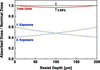Figure 1 Dose distribution in a 200 µm-thick PMMA sample after exposure from the front side (dotted line 1. Exposure') and exposure from the back side (dotted line 2. Exposure') as well as superposition to a homogeneous dose value (solid line). The homogeneity is better than 96%.

To systematically study the dose dependency, we excluded all other parameters in these experiments, only varying the dose: PMMA was used as the resist. A non-crosslinked commercial grade with an initial weight-averaged molecular weight of approximately 1.6 × 106 g mol−1, Acrylite cell cast GP 0F00 from Evonik, was fly-cut to foils of 200 µm and 100 µm thickness. The samples were exposed at the SyLMAND beamline, Canadian Light Source (2.9 GeV electron energy, 16.91 m sample distance from source point, 110 µm total thickness of beryllium vacuum windows, 381 µm Kapton preabsorber for 200 µm-thick samples and 254 µm Kapton preabsorber for 100 µm-thick samples). Unlike for normal exposures, the resist was not attached to a substrate but rather held in a Kapton bag. This allows the sample to be rotated after 50% of the exposure, irradiating the sample from the back side for the second half. Fig. 1illustrates how the dose deposition of both exposures superposes. The sample is also exposed in the absence of a mask (flood exposure'). The total dose deposition anywhere in the foil therefore is almost constant, with an error of only 3.85%.

The dose homogeneity is independent of the exposure time. By varying the integrated electron current, samples with different nominal dose values were exposed under otherwise identical conditions. Lex-D was applied to determine the integrated electron current to obtain dose values between 1 kJ cm−3 and 6 kJ cm−3 for white light (i.e. in the absence of mirrors) as well as for grazing-incidence angles of 6 mrad, 9 mrad and 12 mrad. For the calculations, ideal' mirror conditions were assumed, i.e. clean chromium surfaces of 0.5 nm roughness.

After exposure, each PMMA foil was dried in a vacuum oven at 50°C to remove any ambient moisture, diced into several pieces of 50 mg to 150 mg, and weighed on a precision laboratory scale. Each of the pieces was then developed for different times. The minimum development time during the experiments was 3 min, and the maximum development time amounted to 126 days, spanning almost five orders of magnitude. Dip development without stirring was performed using the so-called GG developer (Ghica & Glashauser, 1982), a mixture of 15 vol% water and three different organic solvents [60 vol% but­oxy­eth­oxy­ethanol (di­ethyl­eneglycolmono­butyl­ether; BDG), 20 vol% morpholine (tetra­hydro-oxazine) and 5 vol% amino­ethanol]. Development was performed at 21°C room temperature. After development, the samples were again vacuum-dried and immediately weighed again. This way, variations of the relative humidity do not impact the mass of the PMMA pieces. The mass reduction during development can be associated with a reduction of the resist foil thickness. We have previously demonstrated (Pantenburg et al., 1998) that under such conditions the reduction of thickness scales linearly with development time, allowing a development rate R (µm min−1) to be defined. We also showed that the exposure dose is the only parameter determining the development rate relative to other samples. The rate can be expressed as a power function (Gipstein et al., 1977),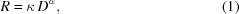with the development rate R (µm min−1), the dose D (kJ cm−3), material parameter κ and resist-developer contrast α. In a log–log plot, a straight line represents the power law of development rate as a function of dose; α is the slope gradient.

Fig. 2exemplarily shows the resist removal as a linear function of development time for the cases of white-light exposure to 2 kJ cm−3, 4 kJ cm−3 and 6 kJ cm−3.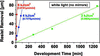Figure 2 Resist removal in 200 µm-thick PMMA foils exposed to white light of 2 kJ cm−3, 4 kJ cm−3 or 6 kJ cm−3, as a function of development time.

The fitted slopes of 0.0604 µm min−1, 0.7375 µm min−1 and 3.9161 µm min−1 constitute three of the four data points for the white-light top curve in Fig. 3. As anticipated, this log–log plot delivers a straight line relationship between development rate and dose deposition. Using equation (1), the development rate amounts to R = 4.0D3.8 µm min−1. The parameter values in the equation are close to those previously found for the same resist and developer material, but a different beamline and spectral distribution (Pantenburg et al., 1998): a rate of R = 3.5D4.0 µm min−1 was determined for the ELSA storage ring in Bonn, Germany. Those experiments were conducted for a slightly higher error in dose homogeneity (8%) and at a lower overall beam power. Both might contribute to the slight offset of the results. Still, development rates agree within a small margin over almost four orders of magnitude. At 1 kJ cm−3, for example, the current study delivers a development rate of 0.0040 µm min−1, which is 14% higher than obtained by Pantenburg et al. (1998). The good overall agreement validates the results of the current study.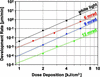Figure 3 Development rates as a function of dose deposition using white light (top curve, 200 µm-thick PMMA foil), and using the double-mirror system set to 6 mrad, 9 mrad or 12 mrad for 100 µm-thick foils. Dose values were determined by Lex-D, assuming ideal' mirror conditions (clean Cr surface, 0.5 nm rough).

#### 2.2. Deduced correction factors for mirror reflectivity

For a fixed set of resist and developer materials and processing conditions, the development rate only depends on the dose. This implies that different white-light spectral distributions should have little or no impact on the overall development rate, as described for our experiments when comparing results obtained at SyLMAND and ELSA, and consistent with the literature (Pantenburg et al., 1998; Gipstein et al., 1977). The same should be true for spectral changes due to mirrors, as long as the dose is properly assessed. Fig. 3shows the fitted lines for the measurements made at 6 mrad, 9 mrad and 12 mrad grazing-incidence angle. Contrary to expectations, the lines do not all coincide. They all have the same slope of 3.66 ± 0.2, within error margins, representing the same resist-developer contrast as expected. However, a vertical offset of the curves corresponds to decreasing development rates at the same nominal doses for increased mirror angles. A physical explanation is that the mirrors have lower actual reflectivities than anticipated, based on calculations using nominal mirror performance values under ideal surface conditions: dose values obtained with the mirrors apparently are overestimated, i.e. actual dose values obtained lag behind theoretical expectation. Dosimetric adjustments for the mirror cases are needed. These adjustments are implemented by determining a correction factor for each mirror angle with the white-light conditions as the reference. Effectively, the white-light development rate curve becomes horizontally shifted to higher dose values until identical development rates are obtained. In a log–log plot, this is mathematically represented by a multiplication factor of the nominal dose. Such a dosimetric correction factor was determined for the three mirror angles. Table 1lists the white-light and three mirror cases, and a selection of key parameters and results: the integrated electron beam current required for a single exposure (either from front or back side) that was simulated with Lex-D to obtain 1 kJ cm−3 nominal dose after double exposure; the development rate parameters according to equation (1); and the nominal dose required for a development rate of 10 µm min−1 as a sample rate. As the four lines in Fig. 3are not exactly parallel, dosimetric correction slightly varies with the nominal dose value analyzed. For further analysis, the development rate obtained for white-light exposure at 10 kJ cm−3 was selected as a reference, because, during normal DXRL exposures, dose values typically range from about 13 kJ cm−3 at the resist top to 3.5 kJ cm−3 at the bottom. At 10 kJ cm−3, the development rate using white light is 26.67 µm min−1. The second to last column in Table 1extrapolates the data plotted in Fig. 3to the nominal dose values required for this same development rate using the mirrors, e.g. 18.3 kJ cm−3 at 6 mrad. In the last column, a dosimetric correction factor is calculated for each mirror setting to obtain this dose value that will deliver the same development rate of 26.67 µm min−1. According to these data, white-light exposure to 10 kJ cm−3 delivers the same development rate as exposure to 18.3 kJ cm−3 using 6 mrad mirrors, to 25.0 kJ cm−3 using 9 mrad mirrors, and to 63.7 kJ cm−3 using 12 mrad mirrors.

 Table 1Required exposure beam currents simulated with Lex-D, and measured development rate parameters for white-light exposure and exposure using the double-mirror system set to 6 mrad, 9 mrad or 12 mrad
 Selected nominal dose values required for different development rates, and correction factors of the nominal dose to obtain the same development rate with mirrors when compared with white-light exposure (at the example of 26.67 µm min−1, or 10 kJ cm−3 white light dose).
Case Integrated beam current for 1 kJ cm−3 (mA min cm−3) Material parameter κ Contrast α Required dose for 10 µm min−1 (kJ cm−3) Required dose for 26.7 µm min−1 (kJ cm−3) Dosimetric correction factor to white light
White light 46 0.003969 3.8275 7.74 10.00 1
6 mrad 127 0.000892 3.5480 13.85 18.26 1.826
9 mrad 291 0.000107 3.8575 19.41 25.04 2.504
12 mrad 1604 0.000018 3.4153 47.77 62.67 6.367

The derived dosimetric correction factors would be independent of the actual dose value if the gradient of the development rates α was exactly identical for all cases, i.e. if the curves in Fig. 3were exactly parallel. The slight differences in gradient result in slightly varying correction factors with dose values. Fig. 4shows error bars for the dosimetric correction factors at 6 mrad, 9 mrad and 12 mrad. Due to the power law governing the development rates, logarithmic values of the correction factors were linearly fitted to obtain an exponential trend line for the correction factors. Fig. 4graphically represents this trend line as a dotted curve according to the equation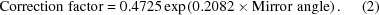From a lithography point of view, certain actual dose values are required to obtain reasonable development rates. The results described indicate that, to obtain those actual dose values, higher nominal dose values are required than simulated under the assumption of ideal mirror conditions. It was derived that the correction factor increases exponentially with the mirror angle. The simulated mirror reflectivity, therefore, is overestimated. Reduced surface density or surface contaminations with oxide or carbon would lead to such reduced reflectivities. The impact of density and contamination is further studied to fit the mirror reflectivity to the results obtained.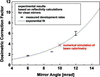Figure 4 Dosimetric correction factor as a function of the mirror angle. Individual points (with error bars) at 6 mrad, 9 mrad and 12 mrad to obtain identical development rates based on measured rates, and fitted curve (dotted line) based on equation (2). The numerical simulation correction data point at 10 mrad was obtained by comparing measured and numerically simulated temperature rises during beam calorimetry at white light and 10 mrad.

### 3. Beam calorimetry

To validate the correction factors deduced from experimental development rate results, a separate experimental technique independent of resist chemistry was applied: beam calorimetry was used to measure the overall beam power. Such an approach has previously been reported to determine beamline performance (Aigeldinger, 2001) and even mirror reflectivity (Nazmov et al., 2008). A small copper block is inserted into the beam, and thermocouples on the back side record the heating of the block as the synchrotron beam is absorbed. Conductive cooling is minimized by hanging the block from thin wires thermally isolated by ceramics or polymers. Convective cooling is insignificant as the exposures are carried out under vacuum conditions. So far, such experiments were performed in a steady state. Absorption of radiation instantly heats up the copper block. As its temperature increases, radiative cooling to the surrounding vacuum chamber (scanner end-station) walls increases, and temperatures eventually come to an equilibrium. Measurement of the maximum temperature is comparatively simple, but deduction of the incident beam power depends strongly on the cooling efficiency, i.e. the emissivity of the copper block and the surrounding walls.

In this study, the impact of emissivity was largely excluded by looking at the transient heating immediately after the start of exposure. During these first seconds, the temperature is almost exclusively determined by the beam power as the copper block is still close to the ambient temperature, and radiative cooling therefore is negligible. A copper block of 3.2 mm thickness can almost completely absorb the incident synchrotron beam. Its lateral dimensions were 4 cm wide by 2 cm high. A chromel/alumel (K-type) thermocouple (5SC-TT-K-40-36 by Omega Engineering; Norwalk, CT, USA) was mounted to the back side of the copper block where it is protected from direct synchrotron radiation. Temperature rises were recorded at a sampling frequency of 8 Hz. Fig. 5shows the temperature rise measured for 10 mrad mirrors and an electron beam current of 204 mA for the first 250 s, as well as a magnified inset for the first 4 s. The linear fit of the temperature rise in the first 4 s delivers a slope of 0.3396 K s−1.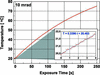Figure 5 Temperature rise in a 3.2 mm-thick copper absorber block upon exposure with 10 mrad mirror angle at 204 mA, SyLMAND beamline, measured with a thermocouple.

Thermal simulations were performed using the software package ANSYS R14.5 to calculate a theoretical temperature gradient on the back side of the copper block when exposed to incident beam on the front side. Lex-D beam power values based on an ideal' mirror surfaces with clean chromium of 0.5 nm roughness were used as input data for the simulations. Finally, measured and simulated temperature gradients for white-light and 10 mrad conditions were compared. The simulated gradient was 3.76 times higher than the measured one, when compared with the white-light reference value. This can be regarded as a reduced mirror reflectivity of the experiments based on the real mirror surface when compared with simulations assuming ideal mirror conditions. This correction factor was implemented in Fig. 4as the numerical simulation' correction factor value (denoted as x) and is in perfect agreement with the correction factor curve based on the development results.

### 4. Reconstruction of mirror surface properties

Table 2lists, for selected mirror angles, the dosimetric correction factors extracted from equation (2)and graphically represented in Fig. 4as obtained from development and beam calorimetry analyses. It also gives the reciprocal values representing the reduced mirror reflectivity compared with ideal' conditions with 0.5 nm rough clean chromium.

 Table 2Dosimetric correction factors extracted from equation (2)and graphically represented in Fig. 4, and reciprocal values representing the correction factors for the postulated reduced mirror reflectivity, for selected mirror angles
Correction factor 6 9 10 12 15 25
Dosimetric 1.65 3.08 3.79 5.75 10.73 86.08
Mirror reflectivity 0.61 0.32 0.26 0.17 0.09 0.01

Chromium density, oxidation and carbon top layer contamination were considered to identify possible combinations that would result in mirror reflectivity corrections consistent with the above mirror reflectivity correction factors.

The overall beam power at the sample location of the SyLMAND beamline was calculated using Lex-D and mirror reflectivity data obtained from Henke et al. (1993). Fig. 6graphically represents the beam power integrated in the spectral range from 0.1 keV to 20 keV photon energies, and normalized to the textbook chromium density of 7.19 g cm−3. The top curve (solid line), for instance, is for 6 mrad mirrors. Even if the chromium density was close to half of the textbook value, at 4.5 g cm−3, the beam power, and therefore the overall mirror reflectivity, would just decrease to 71.1%, while a correction factor of 61% was postulated according to Table 2. For steeper angles, the required reflectivity reductions are even further off from those that could be explained by a reduced chromium density. It is therefore obvious that significant oxidation or contamination must contribute to explain the observed reduction in reflectivity. Those surface layers are likely to mask any density impact, and further calculations are based on the textbook density of 7.19 g cm−3.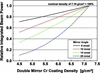Figure 6 Integrated beam power, relative to the textbook chromium density, as a function of the chromium density. Values represent possible reductions in mirror reflectivity compared with the ideal' surface (0.5 nm rough chromium with a density of 7.19 g cm3).

In a systematic study for mirror angles of 6 mrad, 9 mrad, 12 mrad and 15 mrad, 171 cases were simulated for Cr2O3 surface oxidation layers of 1 nm to 500 nm thickness and surface roughness values of 0.5 nm to 8 nm, for carbon contamination layers of 50 nm to 30 µm thickness and surface roughness values of 0.5 nm to 25 nm, as well as a double coating of oxide and carbon.

Fig. 7shows the simulated relative beam power as a function of the layer thickness, at the example of the double mirrors set to 12 mrad. The power values are normalized to the ideal' case of a clean chromium surface with 0.5 nm roughness. The relative beam power therefore also represents the modification of mirror reflectivities relative to the ideal' case assumed in Lex-D. The target correction factor for 12 mrad, as taken from Table 2, is 0.17.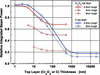Figure 7 Simulated integrated beam power, relative to the ideal' case of a clean chromium surface with 0.5 nm roughness, at the example of 12 mrad mirrors. The relative beam power corresponds to a modified mirror reflectivity (target value of 0.17, cf. Table 2). Three curves for Cr2O3 surfaces of 0.5 nm, 4 nm or 8 nm roughness and a minimum thickness as determined by the roughness, up to 500 nm. Two curves for a carbon contamination layer (0.5 nm or 8 nm roughness) between the thickness corresponding to the roughness value and 30 µm thickness. Spectrally integrated power values compiled for 1 cm beam width at the sample location and an electron current of 250 mA, for the spectral range of 0.1 keV to 20 keV photons, and a vertical acceptance angle limited by 3 mm vertical slit height upstream of the mirrors, at 10.372 m from the source point.

For most cases, the mirror reflectivity is significantly reduced. For thin and smooth coatings, however, the overall reflectivity can even slightly increase above the ideal' case, as previously reported by Lumb et al. (2007). In the case of an oxide layer, the reflectivity strongly decreases with increasing roughness. It also decreases with increasing layer thickness, up to a saturation value reached at 100 nm. A reflectivity reduction to 17% cannot be achieved with an oxide layer alone, within the cases simulated. Based on results by Nazmov et al. (2008), it is unlikely to assume that the oxide layer roughness would reach 8 nm or even go beyond, and simulations beyond the cases studied are not considered reasonable. In the case of a carbon top coat, approximately 1 µm thickness and 8 nm roughness would be required. At 6 mrad, a target reflectivity correction of 0.61 would be reached for roughly comparable contamination properties. Based on previous publications (Boller et al., 1983; Lumb et al., 2007; Dolgov et al., 2015), however, it is assumed that the actual carbon layer thickness would likely be much thinner and smoother.

In subsequent simulations, it was therefore assumed that the mirror oxidized during installation. At 6 mrad, the effect of an oxide layer saturates at 25 nm thickness. This is also approximately the thickness derived by Nazmov et al. (2008) under comparable load. An oxide layer of 25 nm thickness and the original roughness of 0.5 nm was therefore assumed to be contaminated with a carbon layer top coat. This top coat was varied between 50 nm and 2000 nm thickness for roughness values between 0.5 nm and 25 nm. Fig. 8shows a selection of the considered permutations for 6 mrad mirrors, along with the 0.61% reflectivity correction factor target.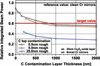Figure 8 Simulated integrated beam power, relative to the ideal' reference case of a clean chromium surface with 0.5 nm roughness, at the example of 6 mrad mirrors. The relative beam power corresponds to a modified mirror reflectivity (target value of 0.61, cf. Table 2). Three curves for a carbon contamination layer (0.5 nm to 25 nm roughness) for thicknesses up to 2000 nm.

The best overall fit between target correction factor and actual correction for all considered angles was obtained for a carbon contamination layer of 50 nm to 100 nm at a roughness of 0.5 nm. In the specific case for 6 mrad illustrated in Fig. 8, this selection would lead to slightly higher reflectivity values than the target value.

To validate the deduced mirror surface properties, the data used for the original development rate experiments were recalculated to correct the input dose values for the non-ideal case. Unfortunately, none of the available DXRL simulation tools allows a three-layer double mirror system with carbon contamination to be modeled. A new simulation tool was therefore developed within this study, and successfully benchmarked with results obtained from Lex-D and DoseSim.

Fig. 9shows the development rates associated with dose deposition values obtained assuming ideal' surface conditions (left graph, equivalent to Fig. 3). The dose values were then corrected simulating a 25 nm-thick and 0.5 nm-rough oxide layer and a 50 nm-thick (center graph) or 100 nm-thick (right graph) carbon top layer of 0.5 nm thickness, and plotted against the measured development rates. In the original case, development rates for white light are up to 568 times faster than for 12 mrad mirrors at nominally the same dose. This error is reduced by more than two orders of magnitude, to a maximum spread of development rates of 4.2 times between white light and 12 mrad mirrors for 50 nm carbon, and 3.0 for 100 nm carbon. At 100 nm carbon layer thickness, the white-light curve is almost centered between the curves for the mirror angles between 6 mrad and 12 mrad, and the least overall error is expected. The remaining error is attributed to the fit to a large but finite number of cases simulated, to the spread of the slopes in the original experiments, and to measurement and simulation inaccuracies accrued during the lengthy process.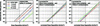Figure 9 Measured development rates as a function of simulated dose deposition using white light and using the double-mirror system set to 6 mrad, 9 mrad or 12 mrad. Left: dose values determined by Lex-D, assuming ideal' mirror conditions (clean Cr surface, 0.5 nm rough). Center: corrected dose values determined with the newly developed simulation tool, assuming a Cr surface, 25 nm Cr2O3 and 50 nm of carbon (all layers 0.5 nm rough). Right: corrected dose values determined with the newly developed simulation tool, assuming a Cr surface, 25 nm Cr2O3 and 100 nm of carbon (all layers 0.5 nm rough).

The adjusted mirror reflectivity for the two cases of 50 nm or 100 nm carbon on 25 nm Cr2O3 also delivers the dosimetric correction factors required at 6 mrad, 9 mrad and 12 mrad angles. Fig. 10repeats the fitted correction curve from Fig. 4, and adds effective correction factors for the 50 nm case (open circles) and the 100 nm case (open diamonds). This last case offers the best overall fit.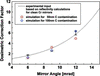Figure 10 Dosimetric correction factor as a function of the mirror angle. Fitted curve for measured development rates and ideal' case dose simulation (dotted line), and correction factors for doses obtained when assuming 25 nm Cr2O3 and 50 nm carbon (open circles) or 100 nm carbon (open diamonds).

The modified mirror surface not only reduces the integrated beam power but also impacts the spectral distribution. Fig. 11depicts the spectral power between 1 keV and 10 keV photon energy for the SyLMAND beamline (110 µm Be vacuum windows, 3 mm slit height) without mirrors (solid curve at top) and for mirrors at 6 mrad. The solid line represents the spectrum for the originally assumed ideal' clean chromium surface without oxide and carbon, while the dashed line illustrates the spectrum in the presence of 25 nm Cr2O3 and 100 nm carbon (all layers with 0.5 nm roughness). The clean Cr surface spectrum shows the abrupt decline in beam power due to the chromium absorption edges between 5.4 and 6.0 keV and the high-energy cut-off between 8 keV and 9 keV. The spectral power of the contaminated surface is marginally increased at photon energies up to 4 keV, due to the increased mirror reflectivity [compare with Fig. 7 of Lumb et al. (2007)]. A pure carbon layer under grazing-incidence exposure at 6 mrad would show a sharp high-energy cut-off at around 5 keV. The spectral response of the carbon-contaminated chromium surface therefore starts to drop at 5 keV. The contamination layer reduces the high-energy cut-off by approximately 2 keV. This value is relatively constant for the simulated angles between 6 mrad and 15 mrad. This emphasizes the necessity for the new DXRL simulation tool developed in this study to reliably predict the dose depth profile in exposed resist.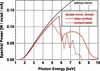Figure 11 Simulated spectral power of the SyLMAND beamline without mirrors and for the chromium-coated double-mirror system at 6 mrad and 0.5 nm roughness (clean Cr surface and 25 nm Cr2O3/100 nm C-contaminated surface).

### 5. Conclusions

We have developed and demonstrated a technique to determine the surface condition of grazing-incidence mirrors exposed to synchrotron radiation. Using this technique, the mirror reflectivity can be assessed without directly probing the surface and without the need for spectral analysis.

PMMA resist foils were homogeneously exposed to dose values between 1 kJ cm−3 and 6 kJ cm−3, for white-light reference samples and for mirror angles between 6 mrad and 12 mrad. The nominal surface condition (0.5 nm rough, clean chromium) was used as input data to determine the exposure parameters. Development rates were measured. Mirror reflectivity correction factors were derived by fitting the development rates for the various cases to the white-light reference results. By varying the chromium density, considering chromium oxidation and a carbon contamination layer, the mirror surface condition was deduced to simultaneously best fit the correction factors for all angles. In the case for the SyLMAND beamline at the Canadian Light Source, the surface properties were derived to be 7.19 g cm−3 nominal chromium density, 0.5 nm roughness for all involved layers, and an oxide layer thickness of 25 nm with a carbon top coat of 50 nm to 100 nm. Those conditions were used as the new input to recalculate the development rate-dose dependency, which now was in good agreement for all mirror angles and the white-light reference.

The developed analysis technique allows an indirect way to qualify a mirror surface. It can be applied at synchrotron beamlines that do not have the space for diffractive analysis or that have too high a flux for energy-dispersive detectors, and that do not have capabilities to probe the mirror surface directly.

Results obtained from such analysis, in combination with the DXRL exposure parameter simulation tool developed, allow the actual DXRL dose deposition to be predicted for any solid material used as vacuum window, filter or mirror surface, and for multiple mirror surface layers. It thus helps to adjust DXRL process conditions to X-ray optical elements in non-ideal conditions or with novel materials, potentially improving the structure quality of fabricated microstructures.

### Acknowledgements

Spectral and beam power calculations have been performed in part by using the Lex-D simulation tool developed by Stewart K. Griffiths and colleagues at Sandia National Laboratories, Livermore, USA.

### ReferencesJOURNAL OFSYNCHROTRONRADIATION